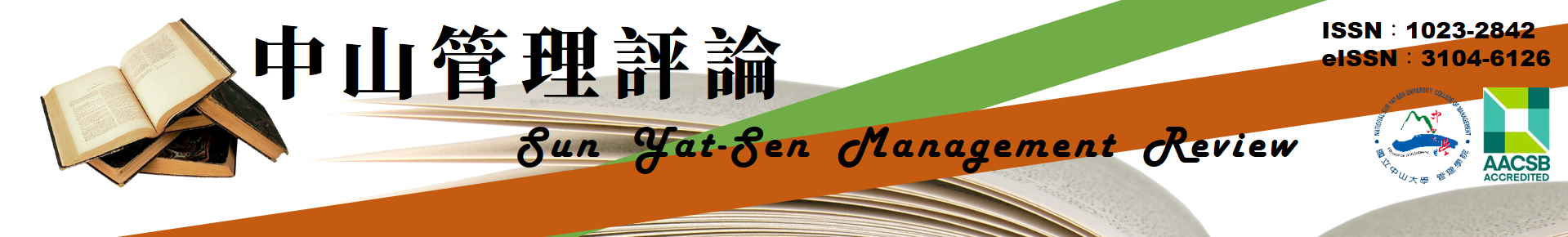### Journal Fullview

Vol. 16, No.4  p.823-850

Title

Controlling Accounting Conservatism and Earnings Growth to Estimate the Implied Cost of Equity Capital
(633719593764601250.pdf 4,125KB)

Author

Huoshu Peng、Mei-Hui Chen/

Department of Accountancy, National Taipei University , Graduate School of Finance, National Defense University

Abstract(Chinese)

(633719593764132500.pdf 9KB)

KeyWord(Chinese)

Abstract(English)

Combining the models of Ohlson (2005) and Dechow & Dichev (2002), this study estimates the implied cost of equity capital by simultaneously controlling the measures of accounting conservatism and earnings growth. When the Capital Asset Pricing Model (CAPM), Arbitrage Pricing Theory (APT), and Fama & French (1993) Three-factor Model got problems in estimating the cost of equity capital, the method based on accounting valuation models may play an important role in this field. The findings show that the model controlling for accounting conservatism and earnings grow exhibit s greater explanatory power to the variation of stock prices than the one without controlling. Furthermore, when comparing the relative explanatory power to the stock returns, there exist no evidence that the accounting-based estimation of the cost of equity capital estimated by the CAPM and future stock returns is inconsistent with the CAPM’s prediction. Further tests of the incremental magnitude between the coefficients of the accounting-based estimation of the cost of equity capital and those estimated by the CAPM, in explaining the variation of future stock returns show that the former dominates the latter, largely because of the negatively significant coefficients of the cost of equity capital estimated by the CAPM.

(633719593764132500.pdf 9KB)

KeyWord(English)

Cost of Equity Capital, Accounting-based Valuation Model, Accounting Conservatism, Earnings Growth

Policy and management implications
(Available only in Chinese)

There are two approaches for the purpose of estimating cost of equity capital. One is the finance-based approach, using models such as Capital Asset Pricing Model (CAPM), Arbitrage Pricing Theory (APT), and Fama & French (1993) Three-factor Model, etc. The other is the accounting-based approach, mainly following the models of Ohlson (1995), Feltham & Ohlson (1995), Ohlson & Juettner-Nauroth (2005), and Ohlson (2005), etc. When the finance-based models got problems in estimating the cost of equity capital, the accounting-based models may play an important role in this field. This study combines the models of Ohlson (2005) and Dechow & Dichev (2002), by simultaneously controlling the measures of accounting conservatism and earnings growth, to estimate the implied cost of equity capital. This approach has the advantage of model completeness relative to previous researches. The findings show that the model controlling for accounting conservatism and earnings growth exhibits greater explanatory power to the variation of stock prices than the one without controlling. Furthermore, when comparing the relative explanatory power to the future stock returns, there exists no evidence that the accounting-based estimation of the cost of equity capital is superior to that estimated by the CAPM. However, the negative relation between the cost of equity capital estimated by the CAPM and future stock returns is inconsistent with the CAPM’s prediction. Further tests of the incremental magnitude between the coefficients of the accounting-based estimation of the cost of equity capital and those estimated by the CAPM in explaining the variation of future stock returns show that the former dominates the latter, largely because of the negatively significant coefficients of the cost of equity capital estimated by the CAPM. The implication to investors is that they should be cautious in using CAPM as a measure of ex ante stock returns.

References